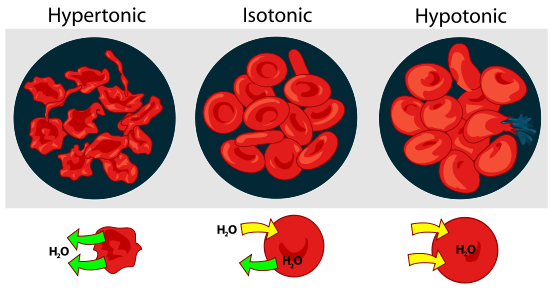# Difference Between Isotonic and Isometric

## Main Difference – Isotonic vs Isometric

Both these terms, Isotonic and Isometric, are useful in chemistry when defining parameters of a chemical reaction or process. The key here is the word ‘iso’. It literally means ‘same’ or ‘similar’. Therefore, it means that the related conditions would remain the same without changing while the process occurs. Tonicity refers to concentration, and, therefore, isotonic would mean having a similar concentration. Metric generally refers to the physical dimensions of an object. And the closest way it could be related to a chemical reaction is through the volume of the container. Therefore, in an isometric process, the volume of the container should remain unchanged throughout the reaction. Therefore, the main difference between isotonic and isometric is that the term isotonic denotes that the concentration of the solution is the same as a reference system whereas isometric denotes that the volume of a reaction stays the same throughout the reaction.

## What is Isotonic

This term is often used in cell biology when comparing the concentration of the cell sap to an external solution. When a solution is isotonic, it is similar in concentration to the cell sap. Therefore, if a cell is placed in this kind of solution, there will be no net transfer of solution to or from the cell. This does not necessarily mean that there is no solution moving out or no solution moving in. It suggests that the rates of the solution moving in and moving out are similar. A normal cell would survive in this kind of an environment.

The process of the solution moving across the cell membrane is known as ‘osmosis.’ And here, the cell membrane acts as a semi-permeable membrane. There are two other kinds of solutions in this case. They are namely hypertonic and hypotonic. Hypertonic is when the concentration of the external solution is greater than that of the cell sap. Here, the solution would start flowing from the cell outwards until the concentration is equal on either side of the cell membrane. A cell, in this case, would suffer dehydration. Hypotonic is when the external solution’s concentration is lower than that of the cell sap. In this case, the solution would flow into the cell and would cause it to swell. Therefore, a cell could only survive when in an isotonic solution.## What is Isometric

In general, when a chemical reaction occurs there is a significant change in the physical properties of the reaction. There are changes in the pressure, volume, and temperature. However, it is also possible to maintain certain properties as a constant while letting the other change. These types of techniques are commonly used when doing research experiments. Here, isometric means when a reaction happens under constant volume. Therefore, in general, the reactions made to occur in a sealed container can be given as an example.

In consideration of the other properties, isothermal means the temperature is maintained constant and isobaric means that the pressure is kept constant.## Difference Between Isotonic and Isometric

### Definition

Isotonic means the concentration of the solution is the same as a reference system.

Isometric is when the volume of a reaction stays the same throughout the reaction.

### Use

Isotonic is usually used in cell biology.

Isometric can be applied to any chemical reaction.

### Principle of Motion

Isotonic involves the physical principle of osmosis.

Isometric doesn’t involve osmosis or any principle of motion.

### Reference

The word isotonic is used to describe a property of a solution.

The word isometric is generally related to space and volume.Image Courtesy:

“Osmotic pressure on blood cells diagram” by LadyofHats – own work based on ,  , and .. (Public Domain) via Commons